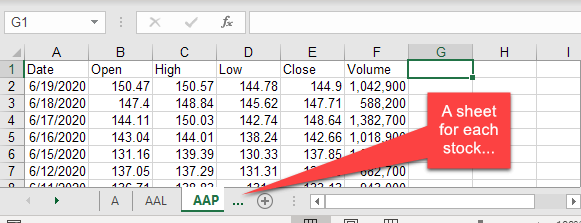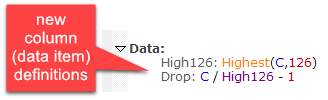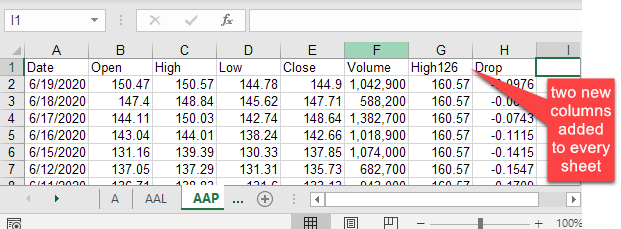Navigation: Realtest Script Language > Script Sections > Data Section

The Data section is probably the most important feature of RealTest. Fully understanding how this section works is critical to your success in using this software.

Say you have just imported daily OHLCV bars for all the stocks in the S&P 500 index, as shown in Tutorial 3.

Imagine that data as sitting in RealTest's active memory, like a virtual Excel Workbook, with a separate Worksheet for each symbol, something like this:Now when you run a script that includes a Data section, here's what happens:

1.Every item defined under Data (in this case High126 and Drop) becomes a new column in EVERY worksheet in this imaginary workbook.

2.The formula provided for each data item is calculated for every bar of every stock and the resulting values are stored in the cells of that column.Now the imaginary workbook looks like this:The two new columns, High126 and Drop, have been added to all 500 worksheets in this imaginary workbook, and for each of those worksheets, values have been calculated and stored for (in the tutorial example) all 1,628 rows of each of these two columns in each of these 500 worksheets. That's 1,628,000 calculations that were made and stored.

All these calculations happen nearly instantly when you run the example scan. In a more complicated multi-strategy trading system model, there will often be billions of calculations to make. Fortunately, RealTest is very fast and takes advantage of all available CPU cores to do this job as quickly as possible.

As a further speed optimization, calculated Data items persist in memory as long as the same data file is in use and their formulas (or the formulas of other items that they refer to) have not been changed. So if, for example, you're running a number of backtests using the same underlying data calculations and only varying, for instance, the exit parameters, that set of tests can run extremely quickly.

To put it another way, calculated data columns are like global variable arrays in a standard programming language. As in an Excel worksheet, once a value has been calculated and stored, it can be referred to in any other formula.

Data column formulas can also refer to their own values that have already been calculated. See Self-Referential Items for details about how that works.

To look "under the hood" at the data columns currently residing in memory, use the Debug Panel.

Another special feature of the data section is the ability to calculate cross-sectional (breadth) data.

Because data columns are pre-calculated before a test is run, they are not allowed to access any test-specific context. If you have a need for intermediate formulas with test-specific context access, that is what the Library Section is for.